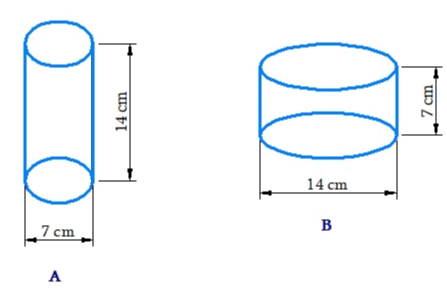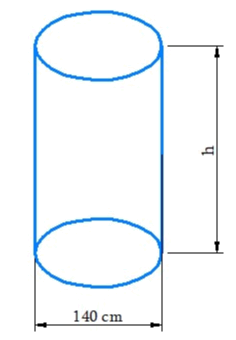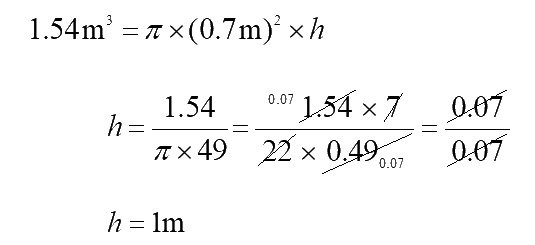# Exercise 11.4 Mensuration- NCERT Solutions Class 8

## Chapter 11 Ex.11.4 Question 1

Given a cylindrical tank, in which situation will you find surface area and in which situation volume.

(a) To find how much it can hold.

(b) Number of cement bags required to plaster it.

(c) To find the number of smaller tanks that can be filled with water from it.

### Solution

What is Known?

Various situations to find surface area and volume.

What is unknown?

Surface area and volume of a cylinder.

Steps:

a) We have to find out the volume of the object.

b) We find area of region covered by a boundary, wall or floor.

c) Volume

## Chapter 11 Ex.11.4 Question 2

Diameter of cylinder $$A$$ is $$7 \rm \,cm,$$ and the height is $$14 \rm\,cm.$$ Diameter of cylinder $$B$$ is $$14 \rm\,cm$$ and height is $$7 \rm\,cm.$$ Without doing any calculations can you suggest whose volume is greater? Verify it by finding the volume of both the cylinders. Check whether the cylinder with greater volume also has greater surface area?### Solution

What is Known?

Dimensions of both cylinder $$A$$ and $$B$$ is given.

What is unknown?

Volume of both the cylinders.

Steps:

From the diagram, radius of cylinder $$A$$ is half of cylinder $$B.$$ so its volume will be lesser than that of cylinder B.

The curved surface area of both cylinders will be same, but the total surface area will be greater in the cylinder with greater radius.

Radius of cylinder

\begin{align} A(r) = \frac{7}{2}\rm\,cm = 3.5\rm\,cm\end{align}

Height of cylinder $$A(h) = 14\rm\,\rm cm$$

Volume of cylinder $$A$$

\begin{align}&=\pi {r^2}h\\& = \frac{{22}}{7} \times 7 \times 7 \times 7\\&= 1078\,\,{\rm{c}}{{\rm{m}}^2}\end{align}

Curved surface area of cylinder $$A$$

\begin{align} &= 2\pi rh\\ &= 2 \times \frac{{22}}{7} \times 3.5 \times 14 = 308\,\,{\rm{c}}{{\rm{m}}^2}\end{align}

Total surface area of cylinder $$A$$

\begin{align}&= \! 2\pi r(r \! + \! h)\\&= \! 2\pi {r^2} \! + \! 2\pi rh\\&= \! \left[ {2 \! \times \! \frac{{22}}{7} \! \times \! 3.5 \! \times \! 3.5} \right]{\rm{c}}{{\rm{m}}^2} \! + \! 308{\rm{c}}{{\rm{m}}^2}\\ &= \! 385\,{\rm{c}}{{\rm{m}}^2}\end{align}

Radius of cylinder

\begin{align}B(r) = \frac{{14}}{2}\,{\rm{cm}} = 7{\rm{cm}}\end{align}

Height of cylinder $${{B}}(h) = 7\rm\,cm$$

Volume of cylinder $$B$$

\begin{align} &=\pi {r^2}h\\ & = \frac{{22}}{7} \times 7 \times 7 \times 7\\ &= 1078\,\,{\rm{c}}{{\rm{m}}^2}\end{align}

Surface area of cylinder $$B$$

\begin {align} &= 2\pi rh\\ &= 2 \times \frac{{22}}{7} \times 7 \times 7\\& = 308\,\,\rm c{m^2}\end{align}

Total surface area of cylinder $$B$$

\begin{align} &= 2\pi r(r + h)\\& = 2\pi {r^2}h + 2\pi rh \\&= (2 \times \frac{{22}}{7} \times 7 \times 7)\,{\rm{c}}{{\rm{m}}^2} + 308\,{\rm{cm}}\\&=308\,{\rm{c}}{{\rm{m}}^2} + 308\,{\rm{c}}{{\rm{m}}^2} = 616\,{\rm{cm}}\end{align}

Thus, volume and surface area of cylinder $$B$$ is greater than cylinder $$A.$$

## Chapter 11 Ex.11.4 Question 3

Find the height of a cuboid whose base area is $$180$$ $${\rm{c}}{{\rm{m}}^2}$$ and volume is $$900$$ $${\rm{c}}{{\rm{m}}^3}$$?

### Solution

What is Known?

Base area and volume of the cuboid is given.

What is unknown?

Height of the cuboid.

Steps:

Base area of cuboid

$= {\rm{length}} \times {\rm{breadth}} = 180\,{\rm{c}}{{\rm{m}}^2}$

Volume of cuboid

$$= {\rm{length \times breadth \times height}}$$

$900{\rm \,m^2} = 180\rm \,c{m^2} \times height$

\begin{align}{\rm{height}} = \frac{{900}}{{180}}\,{\rm{cm}} = 5\,{\rm{cm}}\end{align}

Thus, the height of the cuboid is $$5\rm\,cm.$$

## Chapter 11 Ex.11.4 Question 4

A cuboid is of dimensions $$60 \rm \,cm × 54 \,cm × 30 \,cm.$$ How many small cubes with side $$6 \rm\,cm$$ can be placed in the given cuboid?

### Solution

What is Known?

Side of the cube and dimensions of the cuboid.

What is unknown?

Number of cubes that to be placed in the given cuboid.

Steps:

Volume of cuboid

\begin{align} & = l \times b \times h \\&= 60\,{\rm{cm}} \times 54\,{\rm{cm}} \times 30\,{\rm{cm}}\\ &= 97200\,{\rm{c}}{{\rm{m}}^2}\end{align}

Side of the cube $$6 \rm\,cm$$

Volume of the cube
\begin{align} &= (6)^3{\rm{c}}{\rm{m}}^3 = 216\,{\rm{cm}}\end{align}

Required number of cubes
\begin{align} &= \frac{{\text{volume of cuboid}}}{\text{volume of the cube}} \\ &= \frac {{97200}}{{216}}\\ &= 450\end{align}

Thus$$, 450$$ cubes can be placed in the given cuboid.

## Chapter 11 Ex.11.4 Question 5

Find the height of the cylinder whose volume is $$1.54{{\rm{m}}^3}$$ and diameter of the base is $$140\rm\, cm\,?$$

### Solution

What is Known?

Volume of the cylinder and diameter of the base of the cylinder is given.

What is unknown?
Height of the cylinder.

Steps:If the height of the cylinder $$= h$$

Radius of the base

\begin{align}(r) = \frac{{140}}{2}\,{\rm{cm}} = 70\,{\rm{cm}}=0.7 \rm\,m\end{align}

Volume of the cylinder $$= \pi {r^2}h$$Thus, height of the cylinder is $$1\rm\,m.$$

## Chapter 11 Ex.11.4 Question 6

A milk tank is in the form of cylinder whose radius is $$1.5 \rm\,m$$ and length is $$7\rm\,m.$$ Find the quantity of milk in liters that can be stored in the tank?

### Solution

What is Known?

Shaped of the milk tank is cylinder and its radius and length is known.

What is unknown?

Quantity milk in liter that can be stored in the tank.

Steps:

Radius of cylinder $$= 1.5\rm\,m$$

Length of cylinder $$(h) = 7\rm\,m$$

Volume of the cylinder

\begin{align} &= \pi {r^2}h\\&= \frac{{22}}{7} \times {(1.5)^2} \times 7\,{{\rm{m}}^3}\\ &= 49.5\,\,{{\rm{m}}^3}\end{align}

$$1$$ cubic meter $$= 1000$$ liters

So, $$49.5$$ cubic meter $$= 49.5 \times 1000 = 44500$$ liters

Therefore $$,49500$$ liters of milk can be stored in the tank.

## Chapter 11 Ex.11.4 Question 7

If each edge of a cube is doubled,

(i) How many times will its surface area increase?

(ii) How many times will its volume increase?

### Solution

What is Known?

Initial surface area and volume of a cube.

What is unknown?

Increased surface area and volume of a cube.

Reasoning:

Whenever sides are doubled in any structure then area becomes four times the original structure and the volume becomes eight times the original structure.

Steps:

It the initial edge of the cube is $$l \rm\,cm.$$

If each edge of the cube is doubled, then it becomes $$2l\rm\, cm.$$

(i) Initial surface area $$=$$ $$6{l^2}$$

New surface area

$\, = 6{(2l)^2} = 6 \times 4{l^2} = 24{l^2}$

Ratio $$=$$ $$6{l^2}:24{l^2}=1:4$$

(ii) Initial volume of the cube $$= \,{l^3}$$

New volume$$\, = {(2l)^3} = 8 \times {l^3}$$

Ratio $$=$$ $${l^3}$$: $$8{l^3}$$$$= 1 :8$$

Thus, the surface area will be increased by four times and volume of the cube will be increased by eight times.

## Chapter 11 Ex.11.4 Question 8

Water is pouring into a cuboidal reservoir at the rate of $$60$$ liters per minute. If the volume of reservoir is $$108{\rm{ }}{{\rm\,{m}}^3}$$, find the number of hours it will take to fill the reservoir.

### Solution

What is Known?

Shape of the reservoir is cuboidal. The rate of pouring water into reservoir is given.

What is unknown?

(i) Increased surface area and volume of a cube.

(ii)How much time it will take to fill the reservoir?

Steps:

Volume of the reservoir

\begin{align} &= 108\rm {m^3}\\ &= 108 \times 1000\,\,{\rm{litres}}\\&= {\rm{ 108000}}\,\,{\rm{litres}}\end{align}

Volume of water flowing into the reservoir in $$1$$ minute $$=$$ $$60\rm\,L.$$

In 1 hour

\begin{align}&=(60 \times 60)\,\,{\text{Litres per hour}}\\&=3600\,\,{\rm{litres}}/{\rm{hour}}\end{align}

Required number of hours

\begin{align} &=\frac{108000}{3600}\,\,\text{hours} \\ &=30\,\,\text{hours}\end{align}

Thus , it will take $$30$$ hours to fill the reservoir.

Instant doubt clearing with Cuemath Advanced Math Program